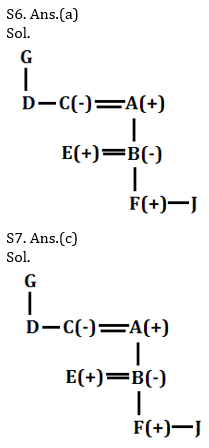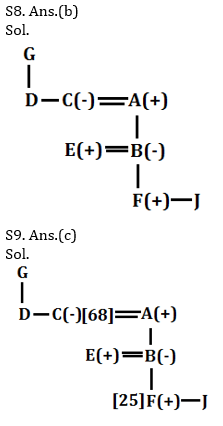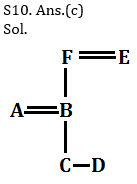Latest Banking jobs   »   Reasoning Ability Quiz For RRB PO...

# Reasoning Ability Quiz For RRB PO Mains 2021- 27th August

Directions (1-5): Study the following information carefully and answer the questions given below:
Ten persons are sitting in a two parallel rows facing each other. A, B, C ,D and E sits in row 1 and faces South. P, Q, R, S and T sit in row 2 and faces north. Each of them have different salaries i.e. 6k, 7k, 8k, 9k, 10k, 12k, 15k, 18k, 19k, 20k but not necessarily in the same order. There are two persons sitting between E and C whose salary is second lowest. A is not facing R and do not earn the highest salary. Q is sitting second to the right of P and is not facing either E or C. R is facing the person whose salary is a multiple of 5. B who is not an immediate neighbor of E is sitting at one of the extreme ends of the line. T who is not facing B and is not getting the highest salary is second to the left of P. Salary of A is double the salary of B but not 18k. S whose salary is 1k lesser than the salary of D is not an immediate neighbor of T and is not getting 19k. Q is getting salary more than S and less than E who is not getting the second highest salary. R is getting a salary of 8k. Difference between the salaries of Q and P is 5k.

Q1. Who is sitting second to the left of the one who is getting 12k?
(a)B
(b)C
(c)E
(d)D
(e)None of these

Q2. Who amongst the following is getting the highest salary?
(a)E
(b)Q
(c)P
(d)Cannot be determined
(e)None of these

Q3. Which of the following is false regarding S?
(a)S is facing C
(b)Salary of S is more than that of B
(c)R is sitting second to the left of S
(d)S is at extreme end of the line
(e)P is an immediate neighbor of S

Q4. Four of the following five are alike in a certain way and belong to a group. Which of the following does not belong to that group?
(a)T-12k
(b)A-19k
(c)B-20k
(d)R-7k
(e)E-8k

Q5. Which of the following is true regarding E?
(a)E is an immediate neighbor of A
(b)E faces immediate neighbor of P
(c) E is getting the highest salary
(d)E is sitting third to the right of C
(e)P is getting lesser salary than E

Directions (6-10): Read the following information carefully and answer the questions given below it.
P% Q means P is sibling of Q
P\$Q means P is parent of Q
P#Q means P is grandparent of Q
P&Q means P is spouse of Q
+P/+Q represents the male members
-P/-Q represents the female members

[Note: consider only given persons in the question]

Q6. If the expression “G\$ D %(-)C &A\$B&(+)E ; C#(+)F%J” holds true , then how is A related to F?
(a) Maternal grandfather
(b) Paternal grandmother
(c) Paternal grandfather
(d) Uncle
(e) None of these

Q7. If the expression “G\$ D %(-)C &A\$B&(+)E ; C#(+)F%J” holds true , then how many married couples are there in the family?
(a) One
(b) Three
(c) Two
(d) None of these
(e) Four

Q8. If the expression “G\$ D %(-)C &A\$B&(+)E ; C#(+)F%J” holds true , then how is A related to G?
(a) Son
(b) Son in law
(c) Brother
(d) None of these
(e) Brother in law

Q9.In the given expressions “G\$ D %(-)C &A\$B&(+)E ; C#(+)F%J” , if the age of C is 68 and the age of F is 25 then what is the possible age of B?
(a) 23
(b) 70
(c) 40
(d) 20
(e) 75

Directions (10): Study the following information carefully and answer the questions given below:
P%Q means P is sibling of Q
P\$Q means P is parent of Q
P#Q means P is grandchild of Q
P@Q means P is child of Q
P&Q means P is spouse of Q

Q10. If the expression “B\$C@A ; D#E&F\$B” hold true, then how C is related to D ?
(a) Brother
(b) Sister
(c) Either (a) or (b)
(d) Mother
(e) Father

Solutions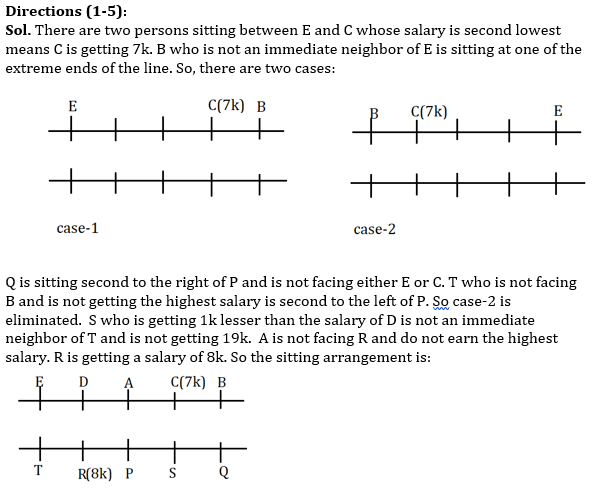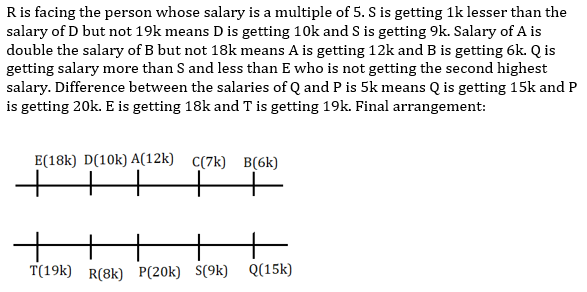S1. Ans (a)

S2. Ans (c)

S3. Ans (d)

S4. Ans (e)

S5. Ans (d)